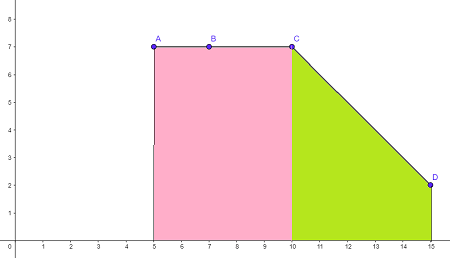# You are given four points in a plane; A= (5,?7), B=(7,7), C=(10,?7), and D=(15,2). The graph of...

## Question:

You are given four points in a plane; A= (5,?7), B=(7,7), C=(10,?7), and D=(15,2). The graph of the function f(x) consists of three line segments AB, BC, and CD. Find the {eq}\int ?155 f(x) dx {/eq} by interpreting the integral in terms of sums and/or differences of areas of elementary figures.

## The Area under the Graph

• If {eq}f(x) \geq 0 {/eq} on {eq}[a,b] {/eq}, then {eq}\int_a^b f(x)dx {/eq} is the area under the graph of {eq}f {/eq} over the interval {eq}[a,b] {/eq}.
• The area of a trapezoid with heights {eq}h_1 {/eq} and {eq}h_2 {/eq} and base {eq}b {/eq} is given by {eq}\displaystyle A = \frac{1}{2}(h_1+h_2)b {/eq}.

Plot the points {eq}A= (5,7), B=(7,7), C=(10,7), {/eq} and {eq}D=(15,2) {/eq}. Draw the line segments {eq}AB, BC, {/eq} and {eq}CD {/eq}. The situation is illustrated in the figure below.The shaded region consists of two subregions: a rectangle (pink) and a trapezoid (green). The area of the rectangle is {eq}A_1 = (5)(7)=35 {/eq}, and the area of the trapezoid is {eq}A_2 = \frac{1}{2}(7+2)(5) = 22.5 {/eq}. The combined area is {eq}A = A_1 + A_2 = 35+22.5 = 57.5 {/eq}. Thus, the integral of {eq}f {/eq} over the interval {eq}[5,15] {/eq} is {eq}\int_{5}^{15} f(x)dx = 57.5 {/eq}.